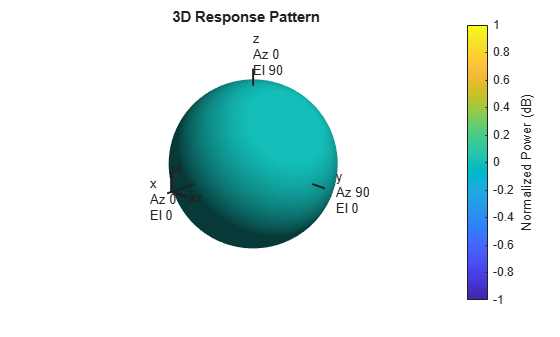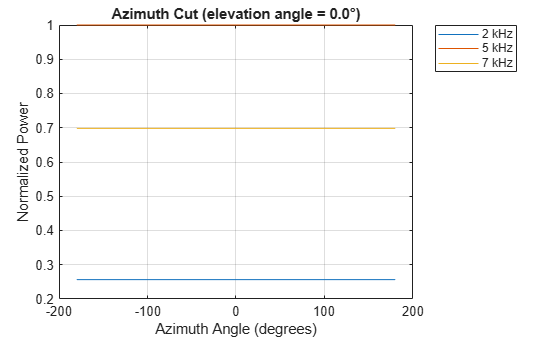# pattern

System object: phased.IsotropicHydrophone
Package: phased

Plot isotropic hydrophone directivity and patterns

## Syntax

```pattern(hydrophone,FREQ) pattern(hydrophone,FREQ,AZ) pattern(hydrophone,FREQ,AZ,EL) pattern(___,Name,Value) [PAT,AZ_ANG,EL_ANG] = pattern(___) ```

## Description

`pattern(hydrophone,FREQ)` plots the 3D directivity pattern (in dBi) for the hydrophone, `hydrophone`. The operating frequency is specified in `FREQ`.

`pattern(hydrophone,FREQ,AZ)` plots the directivity pattern at the specified azimuth angle.

`pattern(hydrophone,FREQ,AZ,EL)` plots the directivity pattern at specified azimuth and elevation angles.

`pattern(___,Name,Value)` plots the directivity pattern with additional options specified by one or more `Name,Value` pair arguments.

`[PAT,AZ_ANG,EL_ANG] = pattern(___)` returns the array pattern in `PAT`. The `AZ_ANG` output contains the coordinate values corresponding to the rows of `PAT`. The `EL_ANG` output contains the coordinate values corresponding to the columns of `PAT`. If the `'CoordinateSystem'` parameter is set to `'uv'`, then `AZ_ANG` contains the U coordinates of the pattern and `EL_ANG` contains the V coordinates of the pattern. Otherwise, they are in angular units in degrees. UV units are dimensionless.

## Input Arguments

expand all

Isotropic hydrophone, specified as a `phased.IsotropicHydrophone` System object.

Example: `phased.IsotropicHydrophone`

Frequencies for computing directivity and patterns, specified as a positive scalar or 1-by-L real-valued row vector. Frequency units are in hertz.

• For an antenna, microphone, or sonar hydrophone or projector element, `FREQ` must lie within the range of values specified by the `FrequencyRange` or `FrequencyVector` property of the element. Otherwise, the element produces no response and the directivity is returned as `–Inf`. Most elements use the `FrequencyRange` property except for `phased.CustomAntennaElement` and `phased.CustomMicrophoneElement`, which use the `FrequencyVector` property.

• For an array of elements, `FREQ` must lie within the frequency range of the elements that make up the array. Otherwise, the array produces no response and the directivity is returned as `–Inf`.

Example: `[1e8 2e6]`

Data Types: `double`

Azimuth angles for computing directivity and pattern, specified as a 1-by-N real-valued row vector where N is the number of azimuth angles. Angle units are in degrees. Azimuth angles must lie between –180° and 180°.

The azimuth angle is the angle between the x-axis and the projection of the direction vector onto the xy plane. When measured from the x-axis toward the y-axis, this angle is positive.

Example: `[-45:2:45]`

Data Types: `double`

Elevation angles for computing directivity and pattern, specified as a 1-by-M real-valued row vector where M is the number of desired elevation directions. Angle units are in degrees. The elevation angle must lie between –90° and 90°.

The elevation angle is the angle between the direction vector and xy-plane. The elevation angle is positive when measured towards the z-axis.

Example: `[-75:1:70]`

Data Types: `double`

### Name-Value Arguments

Specify optional comma-separated pairs of `Name,Value` arguments. `Name` is the argument name and `Value` is the corresponding value. `Name` must appear inside quotes. You can specify several name and value pair arguments in any order as `Name1,Value1,...,NameN,ValueN`.

Plotting coordinate system of the pattern, specified as the comma-separated pair consisting of `'CoordinateSystem'` and one of `'polar'`, `'rectangular'`, or `'uv'`. When `'CoordinateSystem'` is set to `'polar'` or `'rectangular'`, the `AZ` and `EL` arguments specify the pattern azimuth and elevation, respectively. `AZ` values must lie between –180° and 180°. `EL` values must lie between –90° and 90°. If `'CoordinateSystem'` is set to `'uv'`, `AZ` and `EL` then specify U and V coordinates, respectively. `AZ` and `EL` must lie between -1 and 1.

Example: `'uv'`

Data Types: `char`

Displayed pattern type, specified as the comma-separated pair consisting of `'Type'` and one of

• `'directivity'` — directivity pattern measured in dBi.

• `'efield'` — field pattern of the sensor or array. For acoustic sensors, the displayed pattern is for the scalar sound field.

• `'power'` — power pattern of the sensor or array defined as the square of the field pattern.

• `'powerdb'` — power pattern converted to dB.

Example: `'powerdb'`

Data Types: `char`

Display normalized pattern, specified as the comma-separated pair consisting of `'Normalize`' and a Boolean. Set this parameter to `true` to display a normalized pattern. This parameter does not apply when you set `'Type'` to `'directivity'`. Directivity patterns are already normalized.

Data Types: `logical`

Plotting style, specified as the comma-separated pair consisting of `'Plotstyle'` and either `'overlay'` or `'waterfall'`. This parameter applies when you specify multiple frequencies in `FREQ` in 2-D plots. You can draw 2-D plots by setting one of the arguments `AZ` or `EL` to a scalar.

Data Types: `char`

Polarized field component to display, specified as the comma-separated pair consisting of 'Polarization' and `'combined'`, `'H'`, or `'V'`. This parameter applies only when the sensors are polarization-capable and when the `'Type'` parameter is not set to `'directivity'`. This table shows the meaning of the display options.

`'Polarization'`Display
`'combined'`Combined H and V polarization components
`'H'`H polarization component
`'V'`V polarization component

Example: `'V'`

Data Types: `char`

## Output Arguments

expand all

Element pattern, returned as an N-by-M real-valued matrix. The pattern is a function of azimuth and elevation. The rows of `PAT` correspond to the azimuth angles in the vector specified by `EL_ANG`. The columns correspond to the elevation angles in the vector specified by `AZ_ANG`.

Azimuth angles for displaying directivity or response pattern, returned as a scalar or 1-by-N real-valued row vector corresponding to the dimension set in `AZ`. The columns of `PAT` correspond to the values in `AZ_ANG`. Units are in degrees.

Elevation angles for displaying directivity or response, returned as a scalar or 1-by-M real-valued row vector corresponding to the dimension set in `EL`. The rows of `PAT` correspond to the values in `EL_ANG`. Units are in degrees.

## Examples

expand all

Examine the response and patterns of an isotropic hydrophone operating between 1 kHz and 10 kHz.

Set up the hydrophone parameters. Obtain the voltage sensitivity at five different elevation angles: ${-30}^{\circ }$, ${-15}^{\circ }$, ${0}^{\circ }$, ${15}^{\circ }$ and ${30}^{\circ }$. All azimuth angles are at ${0}^{\circ }$. The sensitivities are computed at the signal frequency of 2 kHz.

```hydrophone = phased.IsotropicHydrophone('FrequencyRange',[1 10]*1e3); fc = 2e3; resp = hydrophone(fc,[0 0 0 0 0;-30 -15 0 15 30]);```

Draw a 3-D plot of the voltage sensitivity.

```pattern(hydrophone,fc,[-180:180],[-90:90],'CoordinateSystem','polar', ... 'Type','powerdb')```Examine the response and patterns of an isotropic hydrophone at three different frequencies. The hydrophone operates between 1 kHz and 10 kHz. Specify the voltage sensitivity as a vector.

Set up the hydrophone parameters and obtain the voltage sensitivity at 45° azimuth and 30° elevation. Compute the sensitivities at the signal frequencies of 2, 5, and 7 kHz.

```hydrophone = phased.IsotropicHydrophone('FrequencyRange',[1 10]*1e3, ... 'VoltageSensitivity',[-100 -90 -100]); fc = [2e3 5e3 7e3]; resp = hydrophone(fc,[45;30])```
```resp = 1×3 14.8051 29.2202 24.4152 ```

Draw a 2-D plot of the voltage sensitivity as a function of azimuth.

```pattern(hydrophone,fc,[-180:180],0,'CoordinateSystem','rectangular',... 'Type','power')```expand all